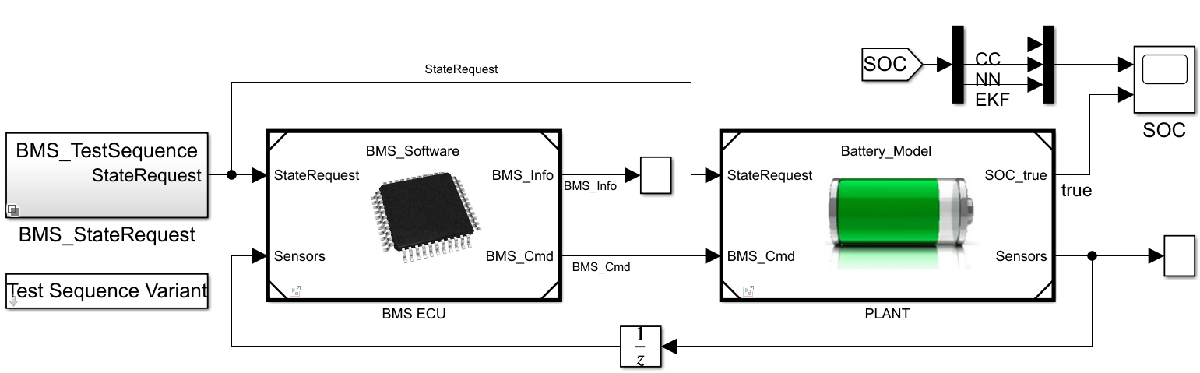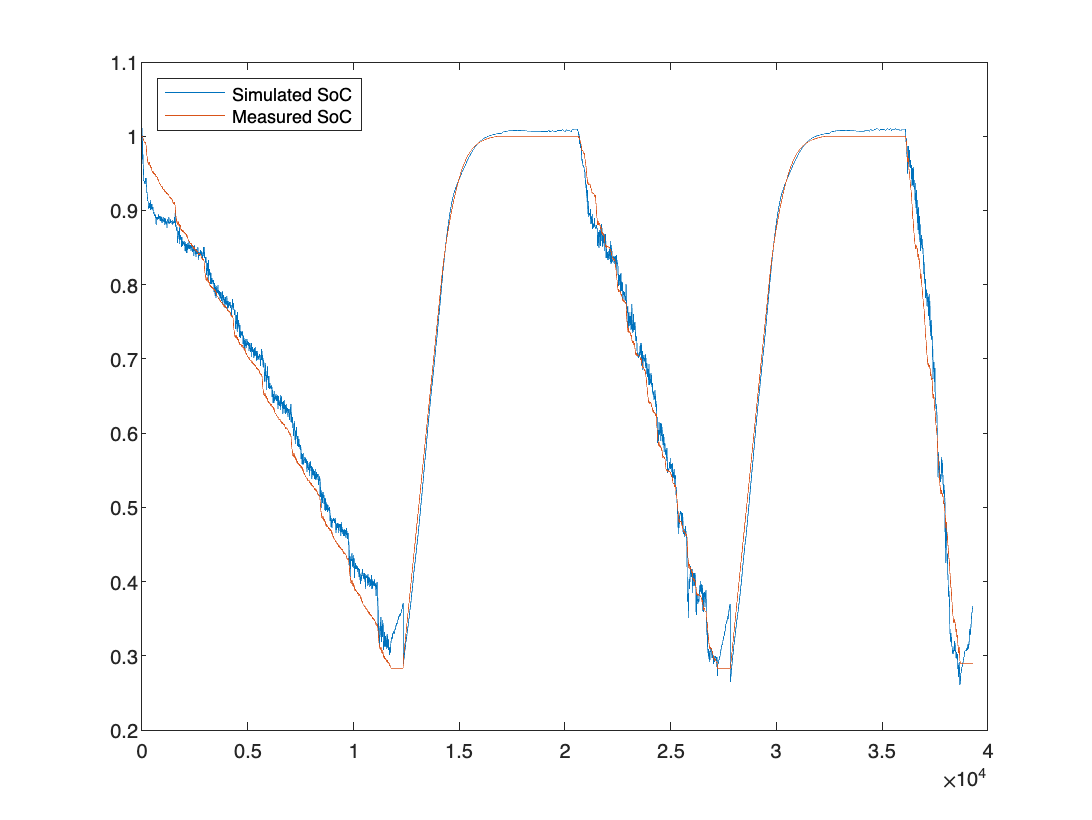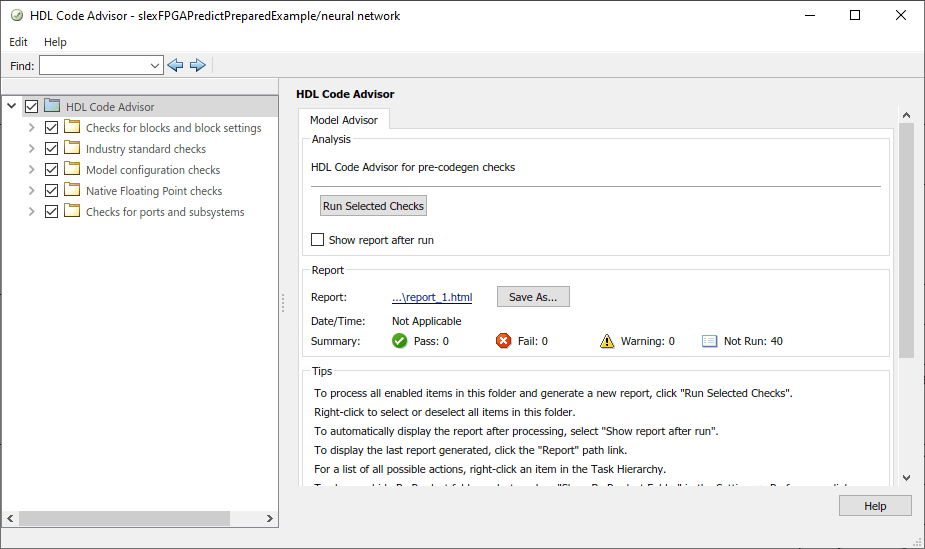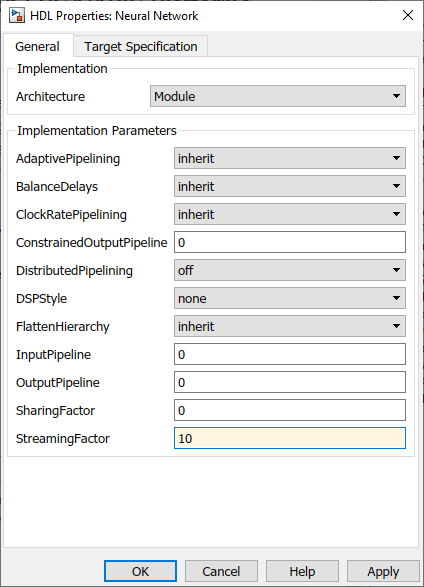# Deploy Neural Network Regression Model to FPGA/ASIC Platform

This example shows how to train a neural network regression model, use the trained regression model in a Simulink® model that estimates the state of charge of a battery, and generate HDL code from the Simulink model for deployment to an FPGA/ASIC (Field-Programmable Gate Array / Application-Specific Integrated Circuit) platform.

State of charge (SoC) is the level of charge of an electric battery relative to its capacity, measured as a percentage. SoC is critical for a vehicle's energy management system. You cannot measure SoC directly; therefore, you must estimate it. The SoC estimation must be accurate to ensure reliable and affordable electrified vehicles (xEV). However, because of the nonlinear temperature, health, and SoC-dependent behavior of Li-ion batteries, SoC estimation remains a significant challenge in automotive engineering. Traditional approaches to this problem, such as electrochemical models, usually require precise parameters and knowledge of the battery composition and physical response.

In contrast, modeling SoC with neural networks is a data-driven approach that requires minimal knowledge of the battery and its nonlinear characteristics . This example uses a neural network regression model to predict SoC from the battery's current, voltage, and temperature measurements .

The Simulink model in this example includes a plant simulation of the battery and a battery management system (BMS). The BMS monitors the battery state, manages the battery temperature, and ensures safe operation. For example, the BMS helps to avoid overcharging and overdischarging. From the battery sensors, the BMS collects information on the current, voltage, and temperature in a closed-loop system.### Train Regression Model at Command Line

To begin, load the data set for this example. Then, train the regression model at the command line and evaluate the model performance.

This example uses the `batterySmall` data set, which is a subset of the data set in . The `batterySmall` data set contains two tables: `trainDataSmall` (training data set) and `testDataSmall` (test data set). Both the training and test data sets have a balanced representation of various temperature ranges. In both data sets, the observations are normalized.

Load the `batterySmall` data set.

`load batterysmall.mat`

Display the first eight rows of the tables `trainDataSmall` and `testDataSmall`.

`head(trainDataSmall)`
``` V I Temp V_avg I_avg Y _______ _______ _______ _______ _______ _______ 0.3855 0.75102 0.49157 0.38535 0.75102 0.20642 0.38704 0.75102 0.85766 0.38571 0.75102 0.20642 0.38709 0.75102 0.85824 0.38572 0.75102 0.20642 0.38924 0.75102 0.85628 0.38658 0.75102 0.20642 0.39174 0.75102 0.90589 0.38919 0.75102 0.20642 0.39338 0.75102 0.90368 0.39149 0.75102 0.20642 0.39508 0.75102 0.91501 0.3939 0.75102 0.20642 0.39529 0.75102 0.91306 0.39417 0.75102 0.20642 ```
`head(testDataSmall)`
``` V I Temp V_avg I_avg Y _______ _______ _________ _______ _______ _______ 0.68309 0.63084 0.0084771 0.8907 0.72464 0.99725 0.74425 0.70388 0.01131 0.87824 0.7214 0.9958 0.76465 0.69525 0.014143 0.85308 0.71765 0.9915 0.91283 0.74702 0.014143 0.86508 0.72354 0.99175 0.81809 0.71665 0.014143 0.86544 0.72384 0.98893 0.63116 0.58937 0.016976 0.86426 0.72321 0.98804 0.55114 0.5736 0.031141 0.7997 0.69442 0.96458 0.90598 0.80312 0.033973 0.79805 0.69475 0.96337 ```

Both tables contain variables of battery sensor data: voltage (`V`), current (`I`), temperature (`Temp`), average voltage (`V_avg`), and average current (`I_avg`). Both tables also contain the state of charge (SoC) variable, which is represented by `Y`.

#### Train Regression Model

Train a neural network regression model by using the `fitrnet` function on the training data set. Specify the sizes of the hidden, fully connected layers in the neural network model.

`nnetMdl = fitrnet(trainDataSmall,"Y",LayerSizes=[10,10]);`

`nnetMdl` is a `RegressionNeuralNetwork` model.

#### Evaluate Model Performance

Cross-validate the trained model using 5-fold cross-validation, and estimate the cross-validated classification accuracy.

```partitionedModel = crossval(nnetMdl,KFold=5); validationAccuracy = 1-kfoldLoss(partitionedModel)```
```validationAccuracy = 0.9994 ```

Calculate the test set accuracy to evaluate how well the trained model generalizes.

`testAccuracy = 1-loss(nnetMdl,testDataSmall,"Y")`
```testAccuracy = 0.9994 ```

The test set accuracy is larger than 99.9%, which confirms that the model does not overfit to the training set.

### Import Model to Simulink for Prediction

This example provides the Simulink model `slexFPGAPredictExample`, which includes the RegressionNeuralNetwork Predict block, for estimating the battery SoC. The model also includes the measured SoC, so you can compare it to the estimated SoC.The `batterySmall` data set contains the `dataLarge` structure with the input data (`X`) and the measured SoC (`Y`). Use the `X` data to create the input data to the `slexFPGAPredictExample` model.

Create an input signal (`input`) in the form of an array for the Simulink model. The first column of the array contains the `timeVector` variable, which includes the points in time at which the observations enter the model. The other five columns of the array contain variables of battery measurements.

```timeVector = (0:length(dataLarge.X)-1)'; input = [timeVector,dataLarge.X]; measuredSOC = [timeVector dataLarge.Y];```

Load the minimum and maximum values of the raw input data used for denormalizing `input`.

```minmaxData = load("MinMaxVectors"); X_MIN = minmaxData.X_MIN; X_MAX = minmaxData.X_MAX; stepSize = 10;```

Open the Simulink model `slexFPGAPredictExample`. Simulate the model and export the simulation output to the workspace.

```open_system("slexFPGAPredictExample.slx") simOut = sim("slexFPGAPredictExample.slx");```

Plot the simulated and measured values of the battery SoC.

```sim_ypred = simOut.yout.get("estim").Values.Data; plot(simOut.tout,sim_ypred) hold on plot(dataLarge.Y) hold off legend("Simulated SoC","Measured SoC",location="northwest")```### Convert Simulink Model to Fixed-Point

To deploy the Simulink model to FPGA or ASIC hardware with no floating-point support, you must convert the RegressionNeuralNetwork Predict block to fixed-point. You can convert the Neural Network subsystem to fixed-point by using the Fixed-Point Tool (Fixed-Point Designer). You can also specify the fixed-point values directly using the Data Type tab of the RegressionNeuralNetwork Predict block dialog box. For more details on how to convert to fixed-point, see Human Activity Recognition Simulink Model for Fixed-Point Deployment.

Open the Simulink model `slexFPGAPredictFixedPointExample`, which is already converted to fixed-point. Simulate the fixed-point Simulink model and export the simulation output to the workspace.

```open_system("slexFPGAPredictFixedPointExample.slx") simOutFixedPoint = sim("slexFPGAPredictFixedPointExample.slx");```

Compare the simulation results for the floating-point (`soc_dl`) and fixed-point (`soc_fp`) estimation of the battery SoC.

```soc_dl_sig = simOut.yout.getElement(1); soc_fp_sig = simOutFixedPoint.yout.getElement(1); soc_dl = soc_dl_sig.Values.Data; soc_fp = soc_fp_sig.Values.Data; max(abs(soc_dl-soc_fp)./soc_dl)```
```ans = 0.0180 ```

This result shows less than a 2% difference between floating-point and fixed-point values for the SoC estimation.

### Prepare Simulink Model for HDL Code Generation

To prepare the RegressionNeuralNetwork Predict block for HDL code generation, you must break the block's link to the Statistics and Machine Learning Toolbox™ library. Doing so allows you to further modify the model.

Navigate to the neural network subsystem, right-click the RegressionNeuralNetwork Predict block, and select Library Link > Disable Link. Right-click the block again and select Library Link > Break Link.

Repeat the same steps to break the library links in the two hidden blocks hiddenLayer1 and hiddenLayer2, which are contained in the RegressionNeuralNetwork Predict block. To see the hidden blocks in the Design section of the Modeling tab, select Model Workspace.Open and run HDL Code Advisor to verify and update the neural network subsystem for compatibility with HDL code generation. For more information, see Check HDL Compatibility of Simulink Model Using HDL Code Advisor (HDL Coder).

Open HDL Code Advisor by right-clicking the neural network subsystem and selecting HDL Code > HDL Code Advisor. Alternatively, you can enter:

```open_system("slexFPGAPredictPreparedExample.slx") hdlcodeadvisor("slexFPGAPredictPreparedExample/neural network")```
```Updating Model Advisor cache... Model Advisor cache updated. For new customizations, to update the cache, use the Advisor.Manager.refresh_customizations method. ```In HDL Code Advisor, the left pane lists the folders in the hierarchy. Each folder represents a group or category of related checks. Expand the folders to see the available checks in each group. Make sure that all the checks are selected in the left pane, and then click Run Selected Checks in the right pane.

If HDL Code Advisor returns a failure or a warning, the corresponding folder is marked accordingly. Expand each group to view the checks that failed. To fix a failure, click Run This Check in the right pane. Then, click Modify Settings. Click Run This Check again after you apply the modified settings. Repeat this process for each failed check in the following lists.

Failed checks in the Checks for blocks and block settings group:

1. Check for infinite and continuous sample time sources

2. Check for large matrix operations - This check flags large matrix operations with Add, Sum, or Product blocks that result in a matrix output with more than 10 elements. These matrix operations can use a large number of resources on the target FPGA. For now, you can skip this check. For more information on efficient implementation of matrix operations, see Optimize the Model for Generating Efficient Code.

Failed checks in the Industry standard checks group:

1. Check clock, reset, and enable signals - This check verifies if the clock, reset, and enable signals follow the recommended naming convention.

2. Check package file names

3. Check signal and port names

4. Check top-level subsystem/port names

Failed checks in the Model configuration checks group:

1. Check for model parameters suited for the HDL code generation

After you apply the suggested settings, run all checks again and inspect that make sure they pass.

#### Replace Blocks with Unsupported Operations

If you try to generate HDL code by right-clicking the neural network subsystem and selecting HDL Code > Generate HDL for Subsystem, the software issues an error about using the Max block to compare scalar values with vectors. The error occurs in these subsystems:

• /neural network/RegressionNeuralNetwork Predict/getScore/hiddenLayers/hiddenLayer1/Activation/relu

• /neural network/RegressionNeuralNetwork Predict/getScore/hiddenLayers/hiddenLayer2/Activation/relu

To fix the issue that causes the error, replace the Max block with the Switch block, as shown in the following figure.### Generate HDL Code

This example provides the Simulink model `slexFPGAPredictReadyExample`, which is ready for HDL code generation. Open the Simulink model.

`open_system("slexFPGAPredictReadyExample.slx")`

To generate HDL code for the neural network subsystem, right-click the subsystem and select HDL Code > Generate HDL for Subsystem. After the code generation is complete, a code generation report opens. The report contains the generated source files and various reports on the efficiency of the code.### Optimize Model for Efficient Resource Usage on Hardware

Open the generated report High-level Resource Report. Note that the Simulink model uses a large number of multipliers and adders/subtractors, because of the matrix-vector operations flagged by HDL Code Advisor. To optimize resource usage, you can enable streaming for your model before generating HDL code. When streaming is enabled, the generated code saves chip area by multiplexing the data over a smaller number of hardware resources. That is, streaming allows some computations to share a hardware resource.

The subsystems that can benefit from streaming are:

• neural network/RegressionNeuralNetwork Predict/getScore/hiddenLayers/hiddenLayer1

• neural network/RegressionNeuralNetwork Predict/getScore/hiddenLayers/hiddenLayer2

To enable streaming for these two subsystems, perform these steps for each subsystem:

1. Right-click the subsystem (hiddenLayer1 or hiddenLayer2) and select HDL Code > HDL Block Properties.

2. In the dialog box that opens, change the StreamingFactor option from 0 to 10, because each hidden layer contains 10 neurons.

3. Click OK.Generate HDL code again and note the reduced number of multipliers and adders/subtractors in the High-level Resource Report. To open the autogenerated version of the model that uses streaming in the generated report, open the Streaming and Sharing report and click the link to the autogenerated model under the link Generated model after the transformation. To see the changes made to the subsystem, navigate to:

/neural network/RegressionNeuralNetwork Predict/getScore/hiddenLayers/hiddenLayer1

To run the autogenerated model, you must extract the parameters of the neural network model that are stored in the mask workspace of the original Simulink model `slexFPGAPredictExample`. These parameters now need to be in the base workspace.

```blockName = "slexFPGAPredictReadyExample/neural network/RegressionNeuralNetwork Predict"; bmw = Simulink.Mask.get(blockName); mv = bmw.getWorkspaceVariables; learnerParams = mv(end).Value;```

### Deploy New Neural Network Model

If you train a new neural network model with different settings (for example, different activation function, number of hidden layers, or size of hidden layers), follow the steps in this example from the start to deploy the new model. The HDL Coder optimization (prior to HDL code generation) might be different, depending on the new model architecture, target hardware, or other requirements.

 Kollmeyer, Phillip, Carlos Vidal, Mina Naguib, and Michael Skells. "LG 18650HG2 Li-ion Battery Data and Example Deep Neural Network xEV SOC Estimator Script." Mendeley 3 (March 2020). https://doi.org/10.17632/CP3473X7XV.3.

 Vezzini, Andrea. "Lithium-Ion Battery Management." In Lithium-Ion Batteries, edited by Gianfranco Pistoia, 345-360. Elsevier, 2014. https://doi.org/10.1016/B978-0-444-59513-3.00015-7.